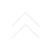#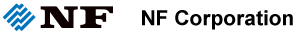Driving the future of technology : Original solution in measurement and control

## Lecture for Measurement

Posted 23/09/2019

### Current Amplifier (Transimpedance Amplifier)

#### 2. Stable Operation Despite Large Capacitance Applied to Input Signal Source

The relationship between input capacitance and stable operation is explained in terms of a current amplifier with a configuration in which current is feedback to the operational amplifier via a resistor (Fig. 4). The equivalent circuit of the input section of this current amplifier is shown in Figure 4.

Figure 4: Feedback-type current amplifier using operational amplifier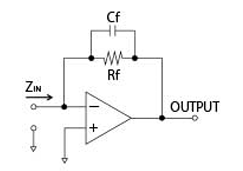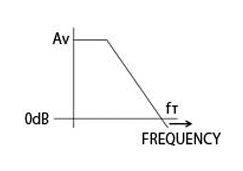Open-loop gain of op amp Gain frequency characteristic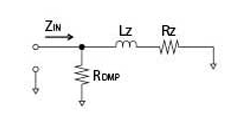Input-section equivalent circuit

If the open-loop gain at DC of the op-amp is Av and the unity gain frequency is f, the constants of the input equivalent circuit are given as follows.

Lz=Rf/(2・π・fт)　(H)
Rz=Rf/(1+Av)　(Ω)
RDMP=1/(2・π・fт・Cf)　(Ω)
Cf is equivalent to damping resistance

In Fig.5, Cs is added in equivalent circuit as capacitance between input to GND. Cs is such as output capacitance of the sensor and the capacitance of the cable from the signal source. As shown in Fig. 5, the equivalent circuit with Cs is a parallel resonant circuit, so operation may be unstable.

Figure 5: Input equivalent circuit with Cs (parallel resonance)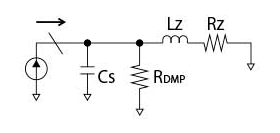In the input-section equivalent circuit in Fig. 5, RDMP (acting as a damping resistor) depends on Cf; therefore, to obtain stability, Cf (corresponding to Cs) is required. Fig.6 shows the gain frequency characteristic when frequency response optimized against Cs (10 pF) is shown in Figure 6. The feedback resistance and signal-source resistance are both 1 GΩ. When Cs is 100 pF, the gain rises slightly near the upper cutoff frequency. And when Cs reaches 1000 pF, the gain rises largely and a peak occurs.

Figure 6: Example of gain frequency characteristics when Cs is added
(optimal adjustment when Rs = Rf = 1 GΩ and Cs = 10 pF)In the above, it was shown that in order to increase the upper cutoff frequency, it is necessary to reduce Cf; however, when capacitance Cs is added to the input, it is necessary to increase Cf to obtain stability. These is a trade-off between stability improvement and upper cutoff frequency (bandwidth).

Due to its circuit design, NF's SA-600/CA-550/CA-650 series is configured so that resonance does not occur even if Cs is applied to the input. Thanks to this configuration, instability due to Cs can be avoided, without large Cf, and wide bandwidth and stable operation are achieved. The gain frequency characteristic of SA-606F2 is shown in Figure 7. Even if Cs is 10 pF, 100 pF, and 1000 pF, it is clear that frequency response is stable.

Typical capacitances of cables and photodiodes equivalent to Cs are listed in Table 4.

Figure 7: Gain-frequency characteristics of SA-606F2Table 4: Capacitances of cables and photodiodes

Product name Parallel capacitance
50-Ω BNC cable About 100 pF/m
75-Ω BNC cable About 67 pF/m
Photodiode (small type) A few pF to 100 pF
Photodiode (large type) About 100 pF to 1000 pF

Related Product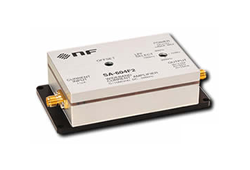Wideband Current Amplifier SA-600 Series

World's top level current amplifier with extremely low noise

Wide current range measurement (sub pico-Amp. to 1 µA)
•  Realize both high gain and wide bandwidth
•  Low noise current density (fA/√Hz order)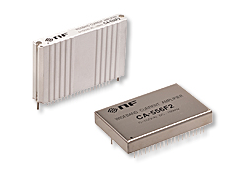Wideband Current Amplifier CA-550 Series / CA-650 Series

CA-550 series and CA-650 series are low noise over wideband current amplifiers with a high gain and wide bandwidth.

Related keywords :  Ultra low noise amplifier, LNA

Recent post